English# FX.co ★ Fractal analysis for major pairs on November 27

### Fractal analysis for major pairs on November 27

Outlook on November 26:

Analytical overview of major pairs on the H1 TF: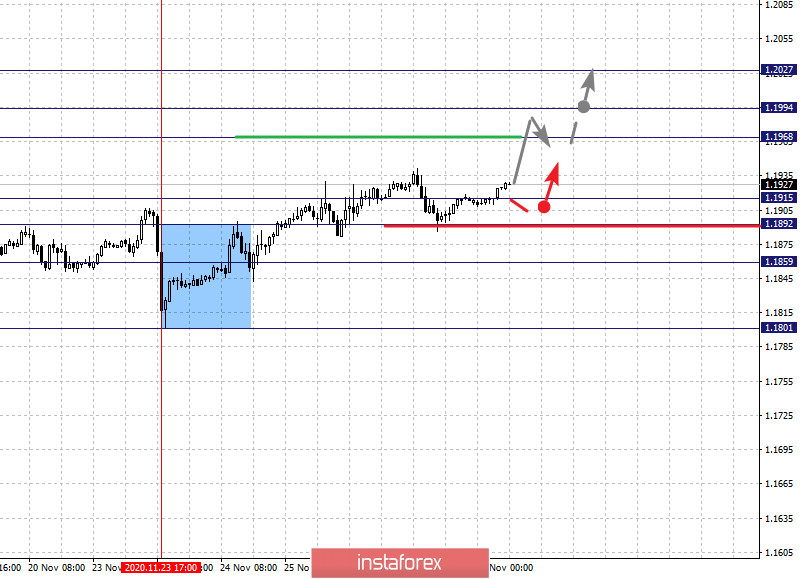The key levels for the euro/dollar pair are 1.2027, 1.1994, 1.1968, 1.1915, 1.1892, 1.1859 and 1.1801. The rising pattern from November 11 is being followed here. We are currently expecting a movement to the level of 1.1968. On the other hand, there is a short-term growth and consolidation in the range of 1.1968 - 1.1994. As an upward potential value, we consider the level of 1.2027. Upon reaching which, downward pullback is expected.

A short-term decline, in turn, is possible in the range of 1.1915 - 1.1892. So, if the last value breaks down, it will lead to a deep correction. The goal here is 1.1859, which is the key upward support level.

The main trend is the local structure of November 23

Sell: 1.1915 Take profit: 1.1892

Sell: 1.1891 Take profit: 1.1860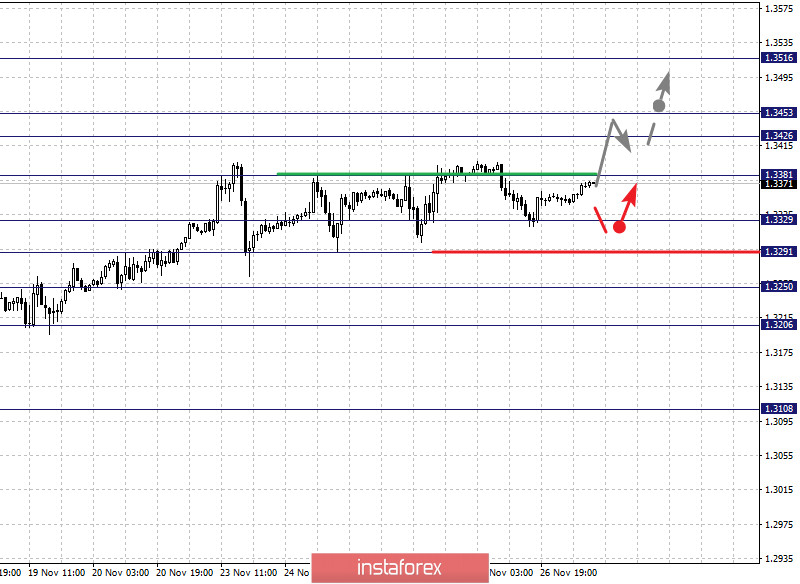The key levels for the pound/dollar pair are 1.3516, 1.3453, 1.3426, 1.3381, 1.3329, 1.3291, 1.3250 and 1.3206. Here, we are following the rising cycle from November 13. The pair is expected to continue rising after the level of 1.3381 breaks down. In this case, the goal is 1.3426. Meanwhile, price consolidation is in the range of 1.3426 - 1.3453. As a potential value for the top, we consider the level 1.3516. A downward pullback is expected upon reaching this level.

On the other hand, a short-term decline is expected in the range of 1.3329 - 1.3291. In case that the last value breaks down, a deep correction will occur. The goal here is 1.3250, which is a key upward support level. If this goal breaks down, it will lead to the development of a downward trend. In this case, the potential goal is 1.3206.

The main trend is the upward structure from November 13

Sell: 1.3329 Take profit: 1.3292

Sell: 1.3289 Take profit: 1.3250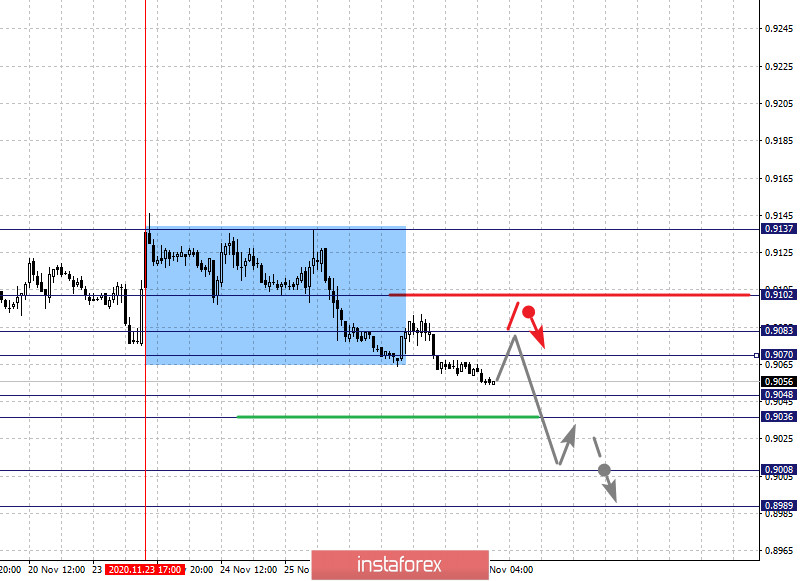The key levels for the dollar/franc pair are 0.9102, 0.9083, 0.9070, 0.9048, 0.9036, 0.9008 and 0.8989. The formation of the descending pattern from November 23 is being monitored here. Now, we expect the pair to continue declining after the price passes the noise range of 0.9048 - 0.9036. In this case, the goal is 0.9008. For the potential value for the bottom, we consider the level of 0.8989. Upon reaching which, price consolidation and upward pullback are expected.

Meanwhile, a short-term growth is expected in the range of 0.9070 - 0.9083. If the last value breaks down, a deep correction will occur. The goal here is 0.9102, which is the key support level for the downside.

The main trend is the initial conditions for the top of November 23

Buy : 0.9070 Take profit: 0.9081

Buy : 0.9084 Take profit: 0.9101

Sell: 0.9034 Take profit: 0.9010

Sell: 0.9007 Take profit: 0.8990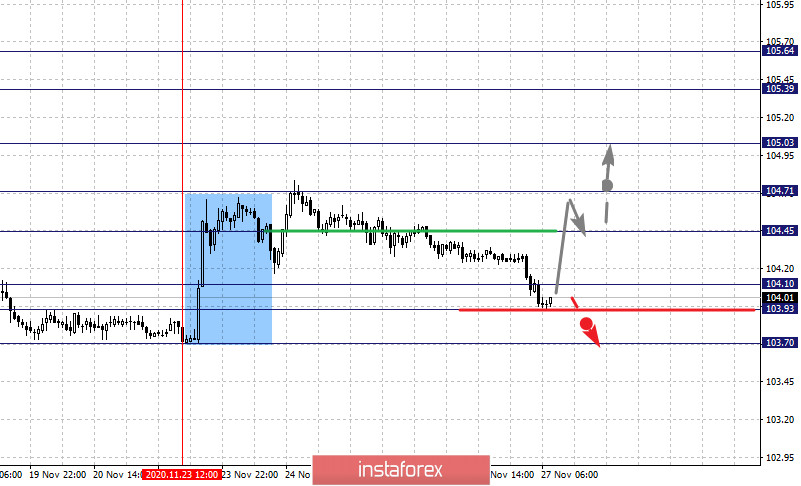The key levels for the dollar/yen are 105.64, 105.39, 105.03, 104.71, 104.45, 104.10, 103.93 and 103.70. Here, price is forming potential initial conditions for the November 23 high and is in deep correction. The upward pattern is expected to be cancelled after the price passes the noise range of 104.10 - 103.93. In this case, the initial goal is 103.70. Now, the pair is expected to rise after the breakdown of 104.45. The first goal here is 104.71. If this level breaks down, we can move to the next key level, that is, the level of 105.03. Price consolidation is near this level.

On the other hand, the breakdown of the level of 105.05 will lead to the development of a strong growth. In this case, the goal is 105.39. For the upward potential value, the level of 105.64 is considered. A downward pullback is expected upon reaching this level.

The main trend is the formation of initial conditions for the upward cycle of November 23, deep correction stage

Buy : 104.72 Take profit: 105.01

Sell: 103.91 Take profit: 103.70

Sell: Take profit: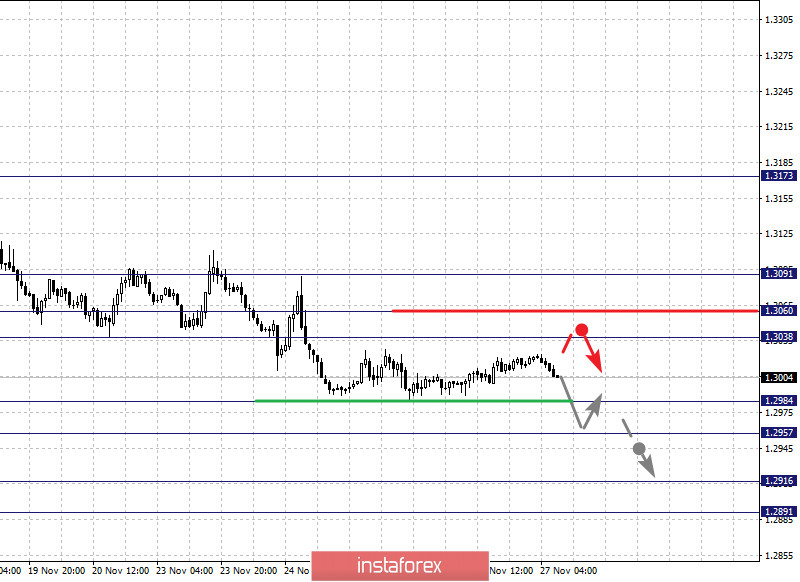The key levels for the USD/CAD pair are 1.3091, 1.3060, 1.3038, 1.2984, 1.2957, 1.2916 and 1.2891. The continuation of the downward pattern from November 13 is being followed here. Now, a short-term decline is expected in the range of 1.2984 - 1.2957. In case that the last value breaks down, it will lead to a strong decline. The goal here will be 1.2916. For the potential value for the bottom, we consider the level of 1.2891. Upon reaching which, price consolidation and upward pullback are expected.

A short-term growth, on the other hand, is likely in the range of 1.3038 - 1.3060. In case that the last value breaks down, a deep correction will occur. Here, the goal is 1.3091, which is a key downward support level.

The main trend is the downward cycle of November 13

Buy : 1.3061 Take profit: 1.3090

Sell: 1.2984 Take profit: 1.2958

Sell: 1.2955 Take profit: 1.2917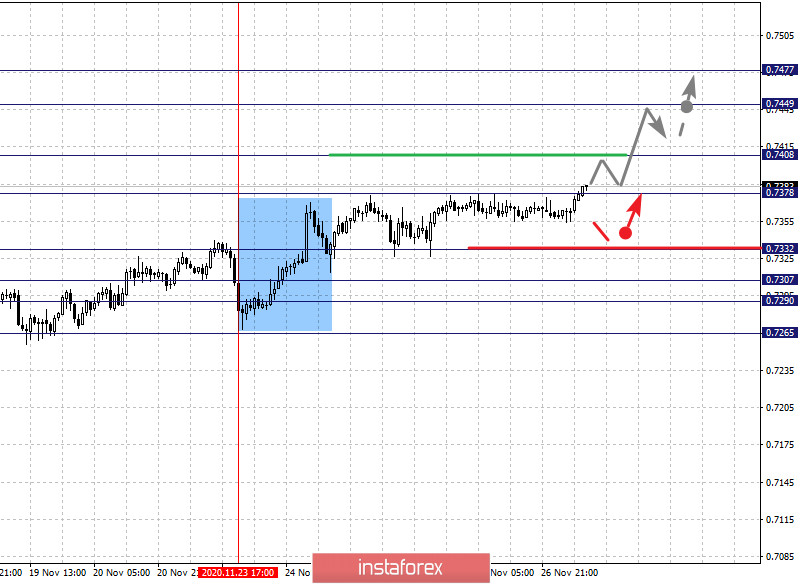The key levels for the AUD/USD pair are 0.7477, 0.7449, 0.7408, 0.7378, 0.7332, 0.7307, 0.7290 and 0.7265. Here, we are monitoring the local bullish pattern from November 23. In view of this, a short-term growth is expected in the range of 0.7378 - 0.7408. If the last value breaks down, it should be accompanied by a strong growth. The goal here is 0.7449. For the potential value for the top, we consider the level of 0.7477. Upon reaching which, price consolidation and downward pullback is expected.

In turn, a short-term decline is expected in the range of 0.7332 - 0.7307, while the key upside support is the range 0.7307 - 0.7290. Thus, the price breaking through this range will lead to the development of a downward trend. In this case, the first potential goal is 0.7265.

The main trend is the local structure of November 23

Sell : 0.7332 Take profit : 0.7308

Sell: 0.7288 Take profit: 0.7265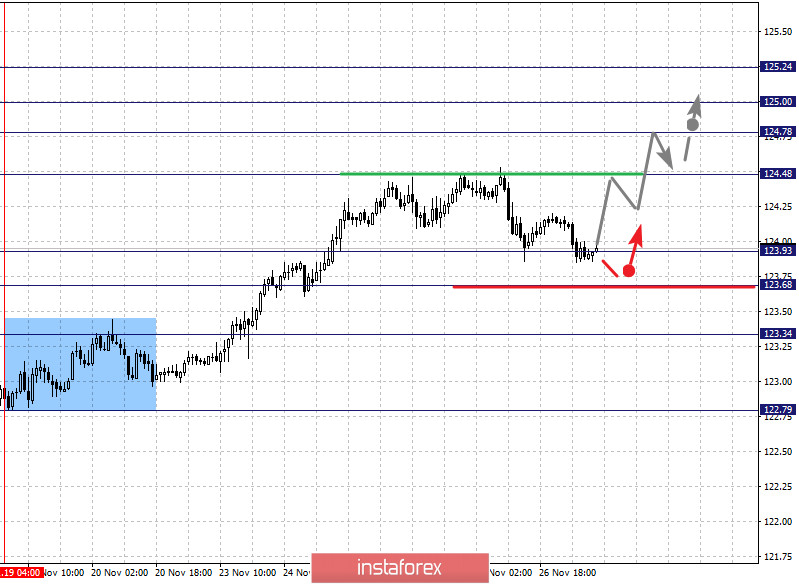The key levels for the euro/yen pair are 125.24, 125.00, 124.78, 124.48, 123.93, 123.68 and 123.34. The upward cycle from November 19 is closely monitored here. Thus, we expect the pair to further rise after the breakdown of the level of 124.48. In this case, the goal is 124.78. On the other hand, there is a short-term growth and consolidation in the range of 124.78 - 125.00. For the upward potential value, we consider the level 125.24. A downward pullback is expected upon reaching this level.

A short-term decline is likely in the range of 123.93 - 123.68. If the last value breaks down, it will lead to a deep correction. The goal here is 123.34, which is the upward key support level.

The main trend is the upward cycle of November 19

Sell: 123.92 Take profit: 123.69

Sell: 123.67 Take profit: 123.36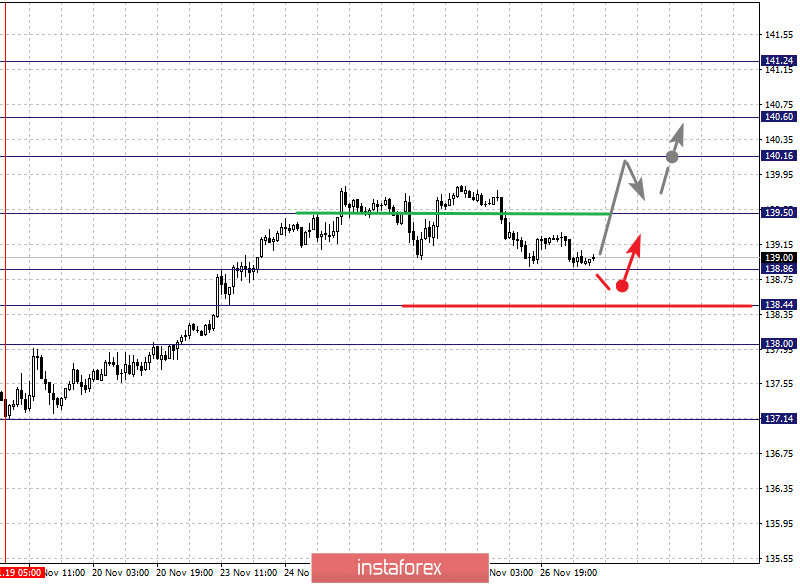The key levels for the pound/yen pair are 141.24, 140.60, 140.16, 139.50, 138.86, 138.44 and 138.00. Here, we are following the formation of the ascending structure from November 19. At the moment, we expect growth to continue after the level of 139.50 breaks down. In this case, the first goal is 140.16. In turn, price consolidation is expected in the range of 140.16 - 140.60. For the potential value for the top, we consider the level 141.24. The downward pullback is expected upon reaching this level.

A short-term decline is possible in the range of 138.86 - 38.44. In case that the last value breaks down, a deep correction will occur. The goal is 138.00, which is the key support for the upside.

The main trend is the upward structure from November 19